# RBSE Maths Class 9 Chapter 5: Important Questions and Solutions

RBSE Maths Chapter 5 – Introduction to Plane Geometry, Line and Angle Class 9 Important questions and solutions are provided here. The important questions and solutions of Chapter 5 available at BYJU’S contain detailed explanations. All these important questions are given based on the new pattern prescribed by the RBSE. Students can also get RBSE Class 9 solutions for all the concepts here.

Chapter 5 of the RBSE Class 9 Maths will help the students to solve problems related to plane geometry. Different axioms are involved in this chapter, which helps the students to understand plane geometry to a greater extent. After practicing these important questions, students will be able to know where to use different concepts related to lines and angles such as vertically opposite angles, linear pair axioms and so on.

### RBSE Maths Chapter 5: Exercise 5.1 Textbook Important Questions and Solutions

Question 1: If angles (2x + 4)° and (x – 1)° form a linear pair, then find out the measure of the angles.

Solution:

Given that the angle (2x + 4)° and (x – 1)° form a linear pair.

That means,

(2x + 4)° + (x – 1)° = 180°

3x + 3° = 180°

3x = 180° – 3°

3x = 177°

x = 177°/3 = 59°

Now,

2x + 4 = 2(59°) + 4° = 118° + 4° = 122°

x – 1 = 59° – 1 = 58°

Therefore, the linear pair of angles are 122° and 58°.

Question 2: From the given figure,

(i) Find the measure of ∠BOD

(ii) Find the measure of ∠AOD

(iii) Which pair of angles have vertically opposite angles?

(iv) Find the adjacent supplementary angles of ∠AOC.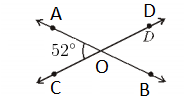Solution:

From the given figure,

(i) ∠AOC = 52°

∠AOC and ∠BOD are vertically opposite angles.

Therefore, ∠BOD = 52°

(ii) ∠AOC and ∠AOD form a linear pair.

By Linear pair axiom,

∠AOC + ∠AOD = 180°

52° + ∠AOD = 180°

∠AOD = 180° – 52°

∠AOD = 128°

(iii) From the given figure, it is clear that there are two sets of linear pair angles.

∠AOC and ∠BOD

∠AOD and ∠BOC

(iv) We know that supplementary angles add up to 180°

Hence, the adjacent supplementary angles of ∠AOC are ∠AOD and ∠BOC.

Question 3: In the figure, OP, OQ, OR and OS are four rays. Prove that:

∠POQ + ∠QOR + ∠SOR + ∠POS = 360°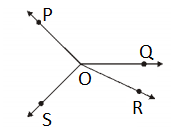Solution:

Extend OQ to get the line OT.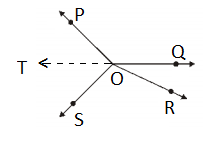Here,

∠POT and ∠POQ form a linear pair.

By Linear pair axiom.

∠POT + ∠POQ = 180° ….(i)

Similarly,

∠TOS, ∠SOR and ∠QOR form a linear pair again.

Thus,

∠TOS + ∠SOR + ∠QOR = 180° ….(ii)

∠POT + ∠POQ + ∠TOS + ∠SOR + ∠QOR = 180° + 180°

∠POT + ∠POQ + ∠TOS + ∠SOR + ∠QOR = 360° ….(iii)

We know that,

∠POT + ∠TOS = ∠POS ….(iv)

From (iii) and (iv),

∠POS + ∠POQ + ∠SOR + ∠QOR = 360°

Hence proved.

### RBSE Maths Chapter 5: Exercise 5.2 Textbook Important Questions and Solutions

Question 4: In the given figure, lines AB, CD and EF are parallel to each other. Find the values of ∠x, ∠y, ∠z and ∠p.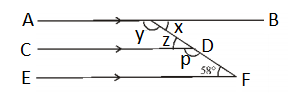Solution:

Given that AB ∥ CD ∥ EF

∠z and 58° are corresponding angles.

Therefore, ∠z = 58°

∠z and ∠x are alternate interior angles.

That means, ∠z = ∠x

Therefore, ∠x = 58°

∠z and ∠p form a linear pair.

By Linear pair axiom,

∠z + ∠p = 180°

58° + ∠p = 180°

∠p = 180° – 58° = 122°

Similarly, ∠x and ∠y also form a linear pair.

∠x + ∠y = 180°

58° + ∠y = 180°

∠y = 180° – 58° = 122°

Hence, ∠p = 122°, ∠x = 25°, ∠y = 122°, ∠z = 58°.

Question 5: In the below figure, AB ∥ EF, find the values of ∠x and ∠y.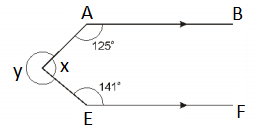Solution:

Given that AB ∥ EF.

∠A = 125

∠E = 141

Draw a line CD which is parallel to the given lines.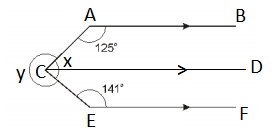∠A and ∠ACD are the interior angles of the same side of the transversal.

∠A + ∠ACD = 180°

125° + ∠ACD = 180°

∠ACD = 180° – 125° = 55°

Similarly,

∠E and ∠DCE are the interior angles of the same side of the transversal.

∠E + ∠DCE = 180°

141° + ∠DCE = 180°

∠DCE = 180° – 141° = 39°

Now, ∠x = ∠ACD + ∠DCE

= 55° + 39° = 94°

We know that, the angle around the point = 360°

∠x + ∠y = 360°

94° + ∠y = 360°

∠y = 360° – 94° = 266°

Therefore, ∠x = 94° and ∠y = 266°.

Question 6: In the below figure, if l ∥ m, then find the angles equivalent to ∠1.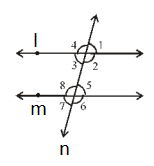Solution:

Given that l ∥ m.

∠1 and ∠5 are the corresponding angles.

∠1 = ∠5

∠1 = ∠3 (vertically opposite angles)

∠1 = ∠7 (alternate exterior angles)

From the above statements,

∠3, ∠5, and ∠7 are the angles equivalent to ∠1.

Question 7: In the below figure, ∠1 = 60° and ∠6 = 120°, then show that m and n are parallel.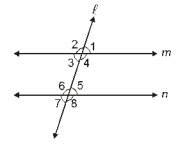Solution:

Given,

∠1 = 60° and ∠6 = 120°

∠1 = ∠3 = 60 (vertically opposite angles)

Now,

∠3 + ∠6 = 60° + 120° = 180°

That means, the sum of the interior angles on the same side of the transversal is equal to 180°

Therefore, the lines m and n are parallel to each other, i.e. m ∥ n.

Question 8: AP and BQ are two bisectors of alternate angles of two parallel lines l and m and its transversal n. Show that AP ∥ BQ.

Solution:

Given that l and m are parallel to each other and n is the transversal.

Also, AP and BQ are the two bisectors of two alternate angles of the parallel lines.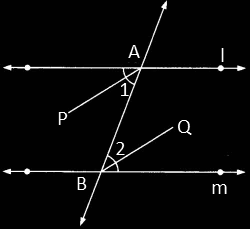∠1 = ∠2 (alternate interior angles)

Thus,

(½) ∠1 = (½) ∠2 (since AP and BQ are bisectors)

(½) ∠PAB = (½) ∠QBA

∠PAB = ∠QBA

Here, the pair of alternate angles formed by the transversal AB which intersects the two lines AP and BQ are equal.

Therefore, AP is parallel to BQ, i.e. AP ∥ BQ.

Question 9: In the figure, BA ∥ ED, then show that: ∠ABC + ∠DEF = 180°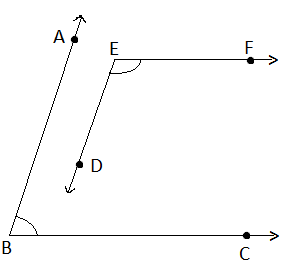Solution:

Given

Let us extend ED to intersect BC.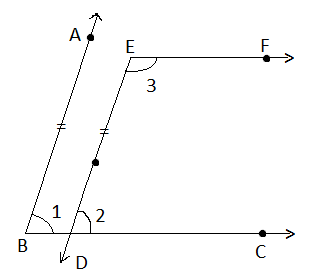Given, BA ∥ ED.

∠1 = ∠2 ….(i)

(corresponding angles)

Also,

BC ∥ EF (from the figure)

Here, the sum of the interior angles on the same side of the transversal is equal to 180°.

∠2 + ∠3 = 180° ….(ii)

From (i) and (ii),

∠1 + ∠3 = 180°

That means,

∠ABC + ∠DEF = 180°

Question 10: In the figure, DE ∥ QR and AP and BP are bisectors of ∠EAB and ∠RBA, then find out the value of ∠APB.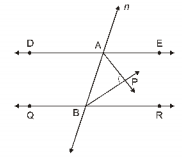Solution:

Given, DE ∥ QR

Here, the sum of the interior angles on the same side of the transversal is equal to 180°.

That means,

∠EAB + ∠RBA = 180°….(i)

Dividing equation (i) by 2,

(1/2)∠EAB + (1/2)∠RBA = 90°….(ii)

Also, given that AP and BP are bisectors of ∠EAB and ∠RBA, respectively.

(1/2)∠EAB = ∠PAB ….(iii)

(1/2)∠RBA = ∠PBA ….(iv)

In triangle APB,

∠PAB + ∠PBA + ∠APB = 180°

Substituting (iii) and (iv),

(1/2)∠EAB + (1/2)∠RBA + ∠APB = 180°

90° + ∠APB = 180°

∠APB = 180° – 90° = 90°

Therefore, ∠APB = 90°

Question 11: If two straight lines are perpendicular to two parallel lines, then show that these straight lines are parallel to each other.

Solution:

Let us draw two parallel lines which are perpendicular to two straight lines.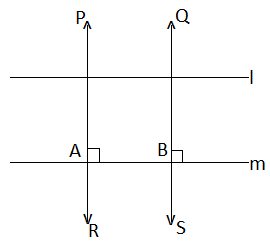PQ and RS are perpendicular to two parallel lines l and m.

∠PAB = 90° and ∠RBA = 90°

∠PAB = ∠RBA = 90°

(corresponding angles)

Therefore, PQ is parallel to RS.

Question 12: In the below figure, if PQ ∥ RS, ∠MXQ = 135° and ∠MYR = 40°, then find ∠XMY.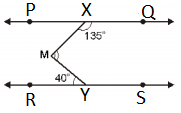Solution:

Given,

PQ ∥ RS, ∠MXQ = 135° and ∠MYR = 40°

Draw a line AB parallel to PQ and RS through M.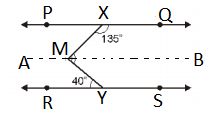AB ∥ PQ

We know that,

Sum of the interior angles on the same side of the transversal is equal to 180°.

135° + ∠XMB = 180°

∠XMB = 180° – 135° = 45° ….(i)

Also,

AB ∥ RS

∠BMY = ∠MYR

∠BMY = 40°….(ii)

(alternate interior angles)

∠XMB + ∠BMY = 45° + 40° = 85°

Therefore, ∠XMY = 85°

### RBSE Maths Chapter 5: Exercise 5.3 Textbook Important Questions and Solutions

Question 13: Draw a line segment AB = 10 cm. Draw its bisector and verify your answer.

Solution:

Steps of construction:

(i) Draw a line segment AB = 10 cm using ruler

(ii) With A and B as centres and radius more than the half of AB, draw arcs on both sides of AB, intersecting each other at P and Q, respectively.

(iii) Join P and Q which intersect AB at M.

(iv) That means M bisects AB.

Therefore, by measuring AM = MB = 5 cm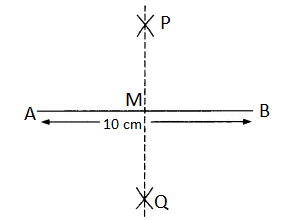Question 14: Draw an angle of 120. Draw a bisector of this angle. Measure both the angles and verify your answer.

Solution:

Steps of construction:

(i) Draw a line AB of any measure.

(ii) With A as the centre, draw an angle of 120° with the help of a protractor, so that ∠DAB = 120°.

(iii) With A as the centre again, draw an arc which intersects the line AB and AD at E and F respectively.

(iv) With E and F as centres and radius more than half of the arc, draw arcs which intersect at P.

(v) Join AP which is the required bisector of ∠DAB.

Therefore, ∠PAB = ∠PAD = 60°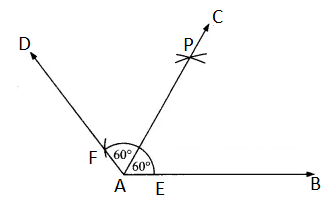Question 15: Draw an angle of 40° with the protractor also draw an angle equal to this angle with ruler and compass.

Solution:

Steps of construction:

(i) Draw ∠BAC = 40° using the protractor.

(ii) Taking A as the centre, draw an arc which intersects AB and AC at M and N, respectively.

(ii) To construct another angle, draw a line segment PQ.

(iii) Take O as the centre on PQ and the radius equal to MN, draw an arc which intersects OQ at R.

(iv) Again, take R as the centres with the same radius draw an arc and mark as S.

(v) Join OS, which is the required angle 40°, i.e. ∠QOS = 40°.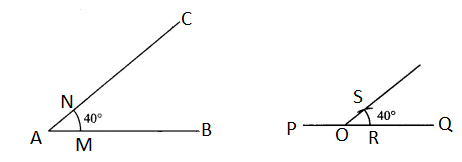Question 16: Draw a line of 6 cm. Draw a perpendicular to it from an outer point P.

Solution:

Steps of construction:

(i) Draw a line segment AB of length 6 cm.

(ii) Take a point P outside the line segment, draw an arc such that it intersects AB at M and N.

(iii) Taking M and N as centres with some radius draw two arcs which intersect at Q.

(iv) Join PQ which intersects AB at O.

Therefore, PQ is perpendicular to AB.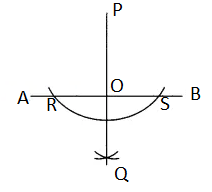Question 17: Draw ∠ABC = 120°. Draw a line parallel to BC from A.

Solution:

Steps of construction:

(i) Draw a line segment BC of some length.

(ii) Taking B as the centre, draw an angle of 120° (∠ABC = 120°) with the help of compass and a ruler.

(iii) From A, draw a line parallel to BC such that the angle at A is 60°

Therefore, AD is parallel to BC.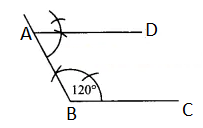Question 18: Draw a line segment of 9 cm. Divide this in three equal parts with ruler and compass.

Solution:

Steps of construction:

(i) Draw a line segment AB of length 9 cm

(ii) Draw a ray of an acute angle at A

(iii) On the ray of acute angle mark three points namely A1, A2 and A3 at equal distances

(iv) Join A3B

(v) Draw line A2Q and A1P which are parallel to A3B

Therefore, AP = PQ = QB = 3 cm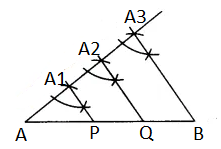Question 19: Draw a line segment of 10 cm. Using a ruler and compass divide it in the ratio 3 : 2. Measure their length.

Solution:

Steps of construction:

(i) Draw a line segment AB of length 9 cm

(ii) Draw a ray of an acute angle at A

(iii) On the ray of acute angle mark five points namely A1, A2, A3, A4 and A5 at equal distances

(iv) Join A5B

(v) Draw line A3P which is parallel to A5B

Therefore, AP = 6 cm and PB = 4 cm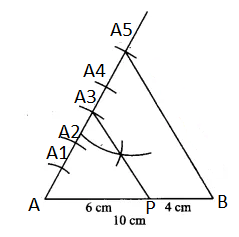Question 20: Draw angle 105° with ruler and compass.

Solution:

Steps of construction:

(i) Draw a ray OA.

(ii) Taking O as centre and with some radius, draw an arc cutting OA at B.

(iii) Taking B as a centre with the same radius, draw an arc which intersects the previous arc at C.

(iv) Now, with C as the centre and with the same radius, draw another arc intersecting the previous arc at D.

(v) Draw a ray OE which passes through C and OF through D.

(vi) Here, ∠AOE = ∠EOF = 60°. Now bisect ∠EOF such that OP is the angle bisector.

(vii) Mark Q where OP intersects the arc.

(viii) Consider C and Q as centres, with the radius more than half of CQ, draw arcs cutting at R.

(ix) Join OR.

Therefore, ∠AOR = 105°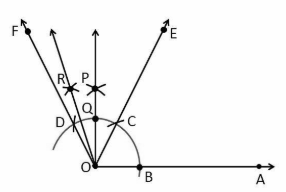### RBSE Maths Chapter 5: Additional Important Questions and Solutions

Question 1: In the figure, if AB ∥ CD ∥ EF, PQ ∥ RS, ∠RQD = 25° and ∠CQP = 60°, then the value of ∠QRS° is equal to: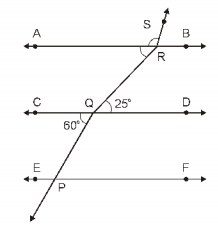1. 85°
2. 135°
3. 145°
4. 110°

Solution:

Given, PQ || RS

∠RQD = 25° and ∠CQP = 60°

∠PQC = ∠BRS = 60°

(alternate exterior angles)

∠DQR = ∠QRA = 25° (alternate interior angles)

∠QRS = ∠QRA + ∠ARS

= ∠QRA + (180° – ∠BRS)

(by linear pair axiom)

= 25° + 180° – 60°

= 205° – 60°

= 145°

Question 2: In the figure, POQ is a straight line. The value of x is: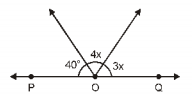1. 20°
2. 25°
3. 30°
4. 35°

Solution:

Given,

POQ is a straight line.

By Linear pair axiom,

40° + 4x + 3x = 180°

7x = 180° – 40°

7x = 140°

x = 140°/7

x = 20°

Question 3: In the below figure, reflex angle AOB is equal to: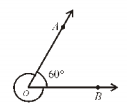1. 60°
2. 120°
3. 300°
4. 360°

Solution:

Given ∠AOB = 60°

We know that the total angle around a point is equal to 360°.

∠AOB + Reflex ∠AOB = 360°

Reflex ∠AOB = 360° – 60° = 300°

Question 4: In the given below figure, two straight lines AB and CD are intersecting each other at point O. The value of ∠x – ∠y is: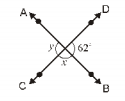1. 56°
2. 118°
3. 62°
4. 180°

Solution:

Given AB and CD are straight lines.

By Linear pair axiom,

62° + ∠x = 180°

∠x = 180° – 62° = 118°

Similarly,

∠x + ∠y = 180°

118° + ∠y = 180°

∠y = 180° – 118° = 62°

∠x – ∠y = 118° – 62° = 56°

Question 5: In the figure, which pair of angles is not a pair of corresponding angles: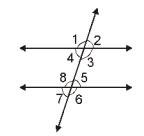1. ∠1, ∠5
2. ∠2, ∠6
3. ∠3, ∠7
4. ∠3, ∠5

Solution:

From the given pairs, except ∠3 and ∠5, remaining pairs are alternate corresponding pair of angles.

Question 6: In the figure, if l and m are two parallel lines which are intersected by a transversal line at points G and H and the angles so formed are shown.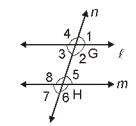If ∠1 is an acute angle, then which statement is false?

(A) ∠1 + ∠2 = 180°

(B) ∠2 + ∠5 = 180°

(C) ∠3 + ∠8 = 180°

(D) ∠2 + ∠6 = 180°

Solution:

∠1, ∠2 are linear pair.

∠2, ∠5 and ∠3 and ∠8 are the corresponding interior angles of same side of the transversal.

Hence, in the above three cases, the sum of two angles is supplementary.

Question 7: From the given figure, find the value of x.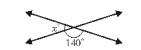Solution:

From the figure,

x and 140 form a linear pair.

By Linear pair axiom,

x + 140° = 180°

x = 180° – 140° = 40°

Question 8: In the figure, AB ∥ CD. Find the value of ∠x and ∠y.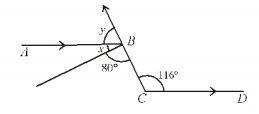Solution:

Given,

AB ∥ CD

∠x + 80° = ∠BCD (alternate angles)

∠x + 80° = 116°

∠x = 116° – 80° = 36°

At the point B,

∠x + ∠y + 80° = 180° (straight line)

36° + ∠y° + 80° = 180°

∠y = 180° – 80° – 36°

∠y = 64°

Question 9: In the figure, lines l and m are parallel. Find the value of ∠x.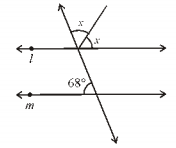Solution: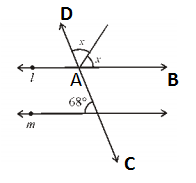∠BAC = 68° (alternate angles)

CD is a straight line.

Thus,

x + x + ∠BAC = 180°

2x + 68° = 180°

2x = 180° – 68°

2x = 112°

x = 112°/2 – 56°

Question 10: In the figure, which lines are parallel and why?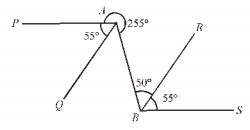Solution:

We know that, if the number of lines meet at a point, then the sum of all the angles so formed is equal to 360 degrees.

From the given figure,

∠PAQ + ∠QAB + Reflex PAB = 360°

55° + ∠QAB + 255° = 360°

∠QAB = 360° – 310° = 50°

∠QAB = ∠ABR = 50° (alternate angles)

QA ∥ BR

Also, ∠PAB = ∠ABS = 105° (alternate angles)

PA BS

Therefore, QA ∥ BR and PA ∥ BS.

Question 11: In the figure, AC ∥ PQ, and AB ∥ RS, then find the value of ∠y. Also, write the statements you have used.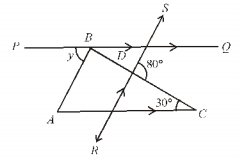Solution: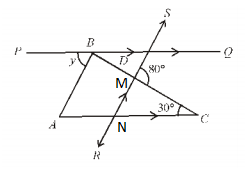Given,

AC ∥ PQ

∠BAC = ∠y….(i)

(alternate angles)

Also, AB ∥ RS

∠BAC = ∠MNC ….(ii)

(corresponding angles)

From (i) and (ii),

∠MNC = ∠y

In triangle MNC,

The exterior angle is equal to the sum of opposite interior angles.

80° = ∠y + 30°

∠y = 80° – 30° = 50°

Question 12: Find the value of x from the diagram if POQ is a straight line.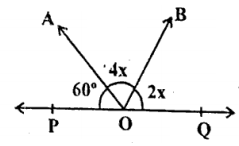Solution:

Given that POQ is a straight line.

By Linear pair axiom,

60° + 4x + 2x = 180°

60° + 6x = 180°

6x = 180° – 60°

6x = 120°

x = 120°/6 = 20°

Question 13: In the given figure, ∠AOC =62°, then find ∠BOC.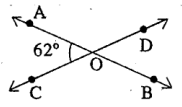Solution:

In the given figure,

∠AOC and ∠BOC form a linear pair.

That means,

∠AOC + ∠BOC = 180°

62° + ∠BOC = 180°

∠BOC = 180° – 62° = 118°

Question 14: Construct an angle of 45° with the help of compass and scale.

Solution: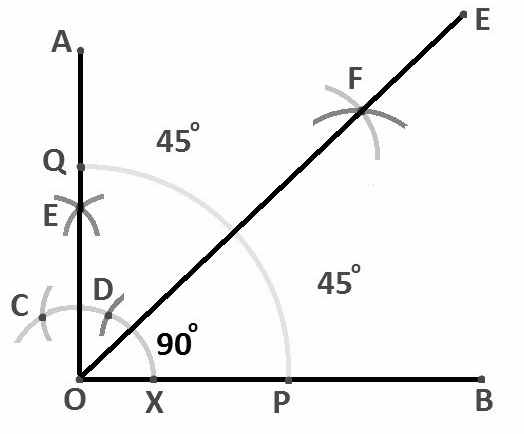Question 15: In the figure lines PQ and RS intersect each other at point O. If ∠POR : ∠ROQ = 4 : 8. Find all angles.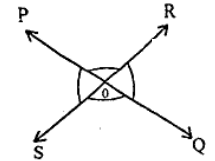Solution:

Given,

∠POR : ∠ROQ = 4 : 8

Let ∠POR = 4x, ∠ROQ = 8x

We know that vertically opposite angles are equal.

∠POQ = ∠SOQ = 4x

∠ROQ = ∠POS = 8x

Sum of the angles around a point is equal to 360 degrees.

∠POQ + ∠SOQ + ∠ROQ + ∠POS = 360°

4x + 4x + 8x + 8x = 360°

24x = 360°

x = 360°/24 = 15°

4x = 4(15°) = 60°

8x = 8(15°) = 120°

Therefore, ∠POQ = ∠SOQ = 60°

∠ROQ = ∠POS = 120°

Question 16: In the figure, find x and y.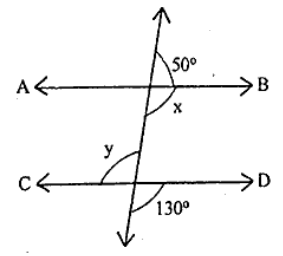Solution:

We know that vertically opposite angles are equal.

Therefore, y = 130°

Also, x and y are alternate angles.

Therefore, x = y = 130°

Question 17: Construct an angle of 135 degrees using a ruler and compass.

Solution:

Steps of construction:

(i) Construct ∠AOD = 90°

(ii) Produce ∠AO to B.

(iii) Draw OE such that it bisects ∠DOB.

Thus,

∠DOE = 45°

∠EOA = 45° + 90° = 135°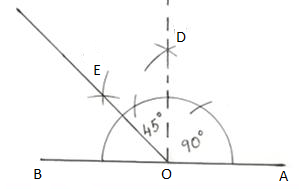Question 18: What is the sum of corresponding angles which are at the same side of the transversal intersecting a pair of parallel lines?

Solution:

The sum of corresponding angles of the transversal which are on the same side to it is equal to 180 degrees.

Question 19: Write Linear pair axiom.

Solution:

If the non-common arms of two adjacent angles form a line, then these angles are called a linear pair of angles.

Question 20: Which of the following pair of angles form a linear pair?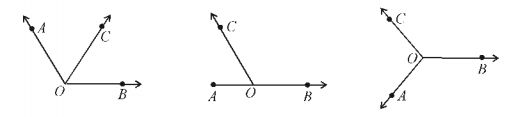Solution:

Angles in the second figure form a linear pair.

Thus, ∠AOC + ∠BOC = 180°

Question 21: If x and y are vertically opposite angles, then:

1. x > y
2. x = y
3. x < y
4. x + y = x – y

Solution:

We know that vertically opposite angles are equal.

Given, x and y are vertically opposite angles.

Hence, x = y

Question 22: In the figure, write the alternate exterior angles.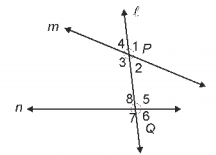Solution:

From the given figure,

Alternate exterior angles are:

∠1 and ∠7

∠4 and ∠6